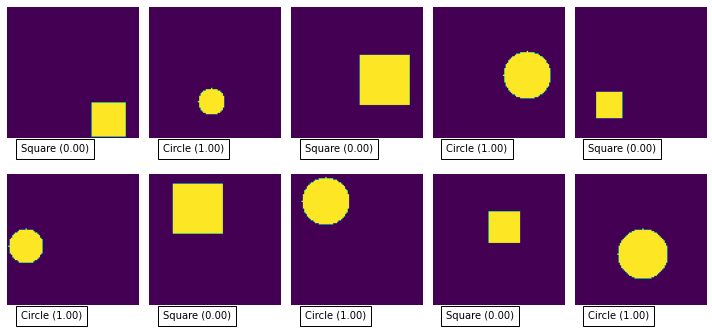read

Binary classification is used where you have data that falls into two possible classes - a classic example would be “hotdog” or “not hotdog” ((if you don’t get the hot dog reference then watch this).

If you’re looking to categorise your input into more than 2 categories then checkout TensorFlow Categorical Classification

You can find the example notebook(s) for this post in the tensorflow-tutorial GitHub repo.

For this small tutorial, I’ve created a python generator that creates images with either a square or a circle.def data_generator():
i = 0
while(True):
if i >= 1000:
i = 0
# our output value will be 0 or 1
Y = i % 2
X = np.zeros((image_width, image_height, 1))

# size of our shape
radius = int(np.random.uniform(10,20))
# position of our shape
center_x = int(np.random.uniform(radius, image_width - radius))
center_y = int(np.random.uniform(radius, image_height - radius))

if Y == 0: # generate a square
X[center_y - radius:center_y + radius, center_x - radius:center_x + radius] = 1
else: # generate a circle
for y in range(-radius, radius):
for x in range(-radius, radius):
if x*x + y*y <= radius*radius:
X[y+center_y, x+center_x] = 1
yield X, [Y]
i = i + 1


Our simple generator will generate an infinite number of samples, alternating between a random square and random circle.

To get binary classification working we need to take note of a couple of things:

1. We need to have one output neuron with a sigmoid activation function. The sigmoid activation function will return a value between 0 and 1 - we’ll use this to determine how confident the network is that input falls the true class.
2. We need to use the (BinaryCrossentropy)[https://www.tensorflow.org/api_docs/python/tf/keras/losses/BinaryCrossentropy] loss function during our training.

Our simple model looks like this:

model = Sequential([
Conv2D(8, 3,
padding='same',
activation='relu',
input_shape=(image_width, image_height, 1),
name='conv_layer'),
MaxPooling2D(name='max_pooling'),
Flatten(),
Dense(
10,
activation='relu',
name='hidden_layer'
),
Dense(1, activation='sigmoid', name='output')
])


And when we compile it we specify the loss function that we want to optimise:

model.compile(optimizer='adam',
loss=tf.keras.losses.BinaryCrossentropy(),
metrics=['accuracy'])


Given our simple problem (is it a square or a triangle) you should be able to get close to 100% accuracy with just a few training epochs.

You can test the model pretty easily by feeding in some more random samples from the training set:

# get a batch of samples from the dataset
X, Y = next(iter(train_dataset))
# ask the model to predict the output for our samples
predicted_Y = model.predict(X.numpy())
# show the images along with the predicted value
plot_images(X, predicted_Y)As you can see it is pretty good at classifying the images, mostly producing 0 or 1 for each image.

Checkout the full code in the GitHub repo.

Image